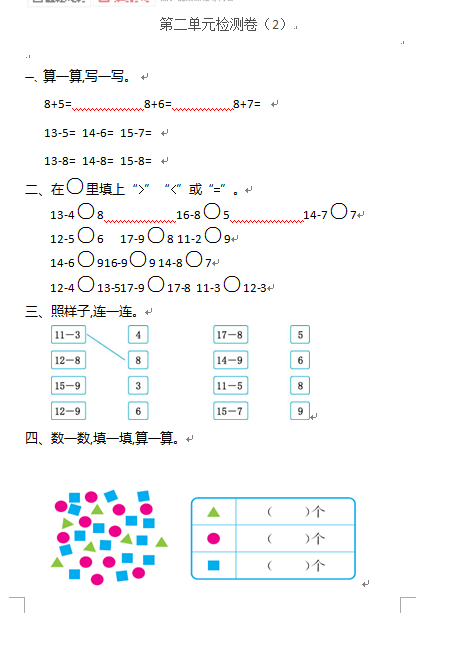8+5=　　　　　　8+6=　　　　　8+7=

13-5=   14-6=   15-7=

13-8=   14-8=   15-8=

【DOC文档4页】小学人教版数学一年级下册第二单元检测卷（2）含答案A4电子版资料_可直接打印_会员免费下载评论信息